Next: The Convergence of the Up: Convergence Theorems in Generalized Previous: Convergence Theorems in Generalized

## The Convergence of a General Value Iteration Process

Let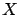be an arbitrary state-space and denote bythe set of value functions over(i.e., the set of bounded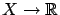functions), and let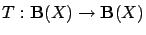be an arbitrary contraction mapping with (unique) fixed point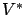.

Let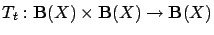be a sequence of stochastic operators. The second argument of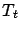is intended to modify the first one, in order to get a better approximation of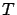. Formally, let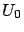be an arbitrary value function and let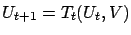.is said to approximateat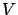with probability one over, if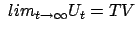uniformly over.

Theorem A.1 (Szepesvári and Littman)   Let the sequence of random operatorsapproximateatwith probability one uniformly over. Let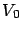be an arbitrary value function, and define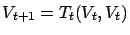. If there exist functions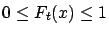and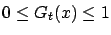satisfying the conditions below with probability one, then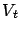converges towith probability one uniformly over:
1. for all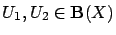and all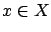,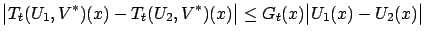2. for all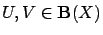and all,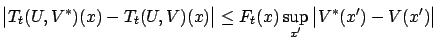3. for all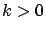,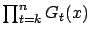converges to zero uniformly inas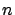increases; and,
4. there exists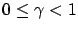such that for alland large enough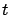,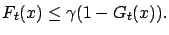The proof can be found in [Szepesvári and Littman(1996)]. We cite here the lemma, which is the base of the proof, since our generalization concerns this lemma.

Lemma A.2   Letbe an arbitrary set,and consider the sequence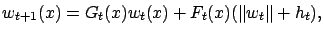where,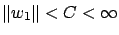with probability one for some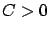,andfor all, and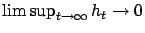w.p.1. Assume that for all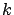,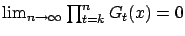uniformly inw.p.1 and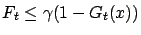w.p.1. Then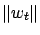converges to 0 w.p.1 as well.Next: The Convergence of the Up: Convergence Theorems in Generalized Previous: Convergence Theorems in Generalized